Like   Tweet   Pin   +1   in

## SEPTEMBER 2017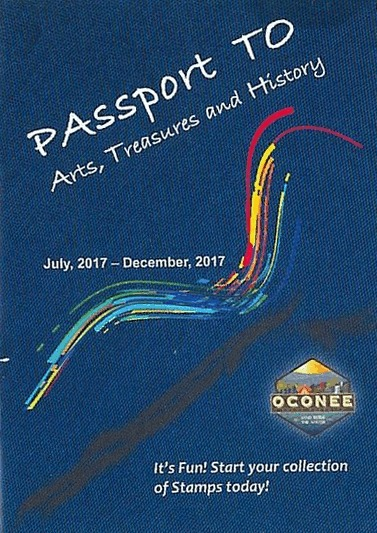Passports are available at the Oconee Chamber of Commerce, Oconee Heritage Center and South Cove County Park.

# Passport to Arts, Treasures and History

The Oconee County Arts & Historical Commission has launched a new program designed to educate and motivate people to tour unique cultural sites throughout Oconee County.

Participants who visit all 21 sites, and take their completed passports to the Parks, Recreation & Tourism (PRT) office at South Cove County Park by the end of 2017, will receive a special gem and be entered for the grand prize treasure, which is a 7-night waterfront camping spot at South Cove Park.

 table div table+table+table+table div table{width:100%;padding:0}table div table+table+table+table div table img{width:96.23%;padding:0;float:none}table div table+table+table+table div table td{width:100%;padding:0 1.88% 18px}/* styles */# "Discover Oconee"

Our podcast episodes feature interesting stories about people and places that make Oconee County a unique destination. Click below to access recent "Discover Oconee" podcasts by our President and CEO Ken Sloan.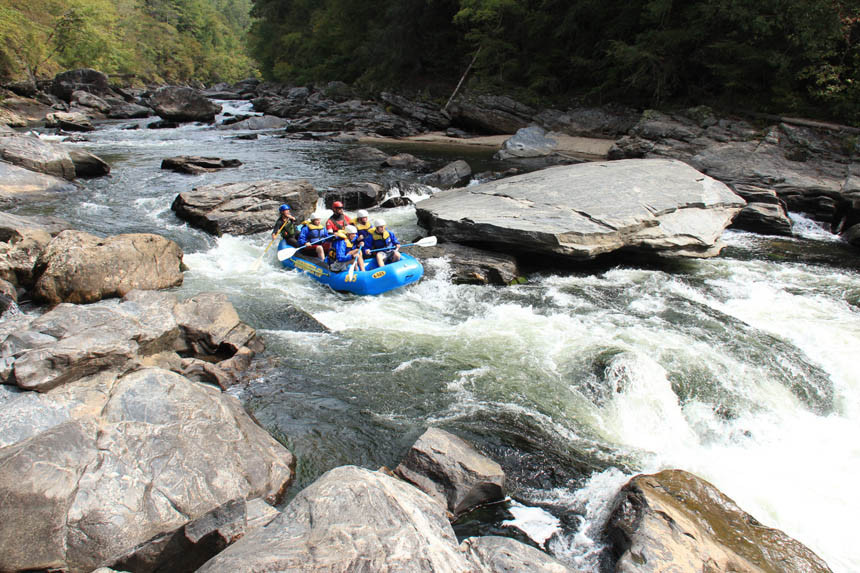EPISODE 4: Rafting & Zipping with Wildwater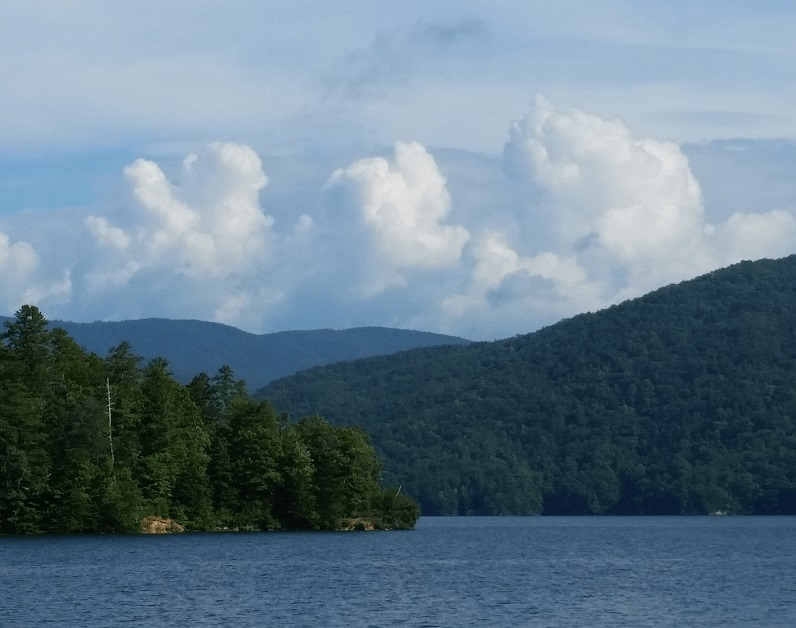EPISODE 5: Natural Wonders with Jocassee Lake Tours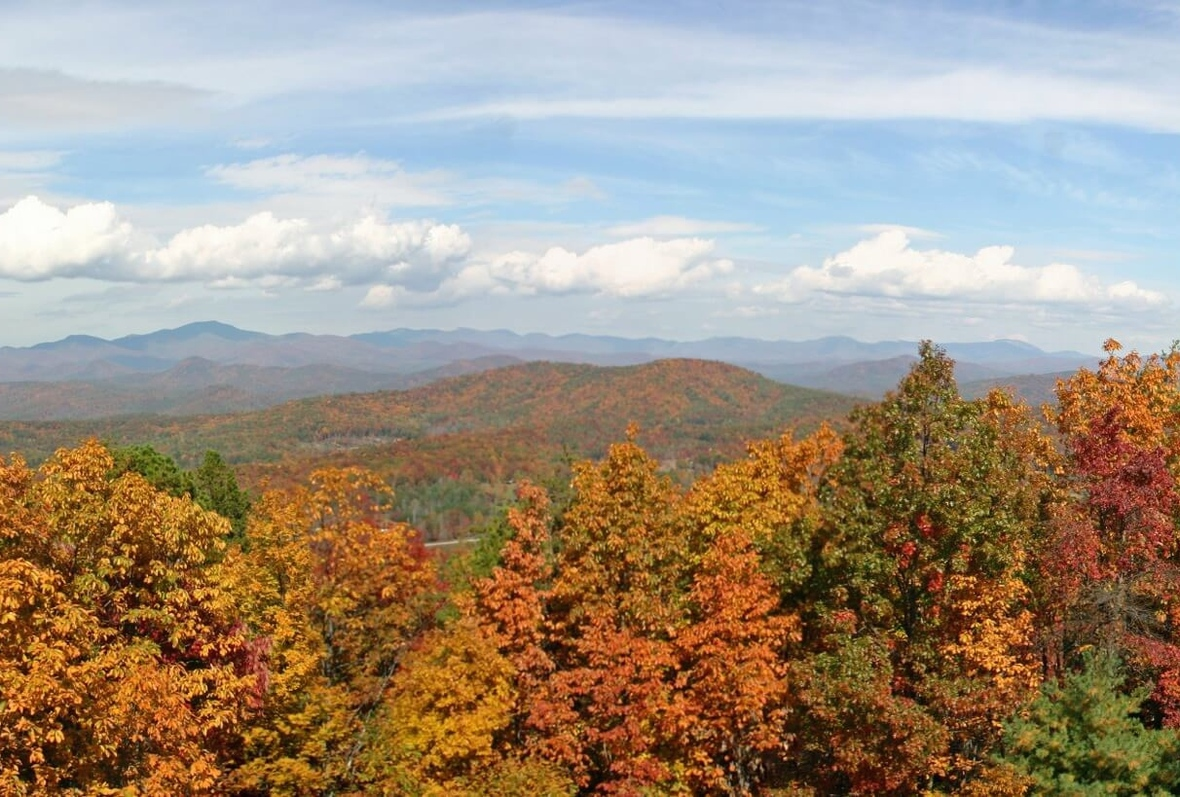EPISODE 6: Making Oconee Even Better with Oconee Economic Alliance & Destination Oconee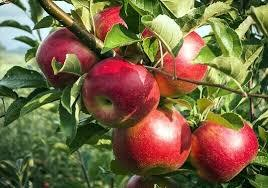Apple varieties grown in Oconee County include: Liberty, Golden Delicious, Pink Lady, Granny Smith, Gale Gala, Ginger Gold, Honey Crisp, Yates, Winesap, Mutsu, Arkansas Black, Fuji, Gibson Golden, Crimson Crisp, Grimes Golden, Hardy Cumberland, Freedom, Gold Rush, Ultima Gala, Pristine, Crimson Gold, Royal Empire, Rome Beauty, Caudle Cameo, and Royal Gala.

# Apples!

Cooler weather means apple season has arrived.
Long Creek and Mountain Rest are located in the westernmost part of Oconee County. This area has an ideal climate for growing apples. At one time, Oconee County was one of the major apple growing areas in the U.S. and had over two dozen growers. Over time imported apples entered the marketplace and government regulations put many growers out of business. Today there are eight orchards in Oconee County and they harvest over 25 varieties of apples.

When you travel through Long Creek and Mountain Rest, make a point to shop at one of the seasonal roadside markets or U-Pick farms. Your support of local growers helps them stay in business and enables them to continue the tradition of cultivating Oconee's picturesque orchards. Visit our website for more details about where to go for apples.

 table div table+table+table+table+table+table+table+table+table div table{width:100%;padding:0}table div table+table+table+table+table+table+table+table+table div table img{width:96.23%;padding:0;float:none}table div table+table+table+table+table+table+table+table+table div table td{width:100%;padding:0 1.88% 18px}/* styles */## FESTIVALS, FAIRS & FOOD

 table.module-10{width:62.64%;padding:0}table div table+table+table+table+table+table+table+table+table+table+table div table{width:62.64%;float:none;margin-left:auto;margin-right:auto;padding:0}table div table+table+table+table+table+table+table+table+table+table+table div table a{border:0 none;text-decoration:none}table div table+table+table+table+table+table+table+table+table+table+table div table img{width:100%!important;border:0 none;text-decoration:none}table div table+table+table+table+table+table+table+table+table+table+table div table td{width:100%;padding:0}/* styles */
 table.module-11{width:89.06%;padding:0}table div table+table+table+table+table+table+table+table+table+table+table+table div table{width:89.06%;float:none;margin-left:auto;margin-right:auto;padding:0}table div table+table+table+table+table+table+table+table+table+table+table+table div table a{border:0 none;text-decoration:none}table div table+table+table+table+table+table+table+table+table+table+table+table div table img{width:100%!important;border:0 none;text-decoration:none}table div table+table+table+table+table+table+table+table+table+table+table+table div table td{width:100%;padding:0}/* styles */
 table.module-12{width:95.47%;padding:0}table div table+table+table+table+table+table+table+table+table+table+table+table+table div table{width:95.47%;float:none;margin-left:auto;margin-right:auto;padding:0}table div table+table+table+table+table+table+table+table+table+table+table+table+table div table a{border:0 none;text-decoration:none}table div table+table+table+table+table+table+table+table+table+table+table+table+table div table img{width:100%!important;border:0 none;text-decoration:none}table div table+table+table+table+table+table+table+table+table+table+table+table+table div table td{width:100%;padding:0}/* styles */
 table.module-13{width:90.94%;padding:0}table div table+table+table+table+table+table+table+table+table+table+table+table+table+table div table{width:90.94%;float:none;margin-left:auto;margin-right:auto;padding:0}table div table+table+table+table+table+table+table+table+table+table+table+table+table+table div table a{border:0 none;text-decoration:none}table div table+table+table+table+table+table+table+table+table+table+table+table+table+table div table img{width:100%!important;border:0 none;text-decoration:none}table div table+table+table+table+table+table+table+table+table+table+table+table+table+table div table td{width:100%;padding:0}/* styles */
 table.module-14{width:90.94%;padding:0}table div table+table+table+table+table+table+table+table+table+table+table+table+table+table+table div table{width:90.94%;float:none;margin-left:auto;margin-right:auto;padding:0}table div table+table+table+table+table+table+table+table+table+table+table+table+table+table+table div table a{border:0 none;text-decoration:none}table div table+table+table+table+table+table+table+table+table+table+table+table+table+table+table div table img{width:100%!important;border:0 none;text-decoration:none}table div table+table+table+table+table+table+table+table+table+table+table+table+table+table+table div table td{width:100%;padding:0}/* styles */table div table+table+table+table+table+table+table+table+table+table+table+table+table+table+table+table+table div table{width:100%;padding:0}table div table+table+table+table+table+table+table+table+table+table+table+table+table+table+table+table+table div table img{width:96.23%;padding:0;float:none}table div table+table+table+table+table+table+table+table+table+table+table+table+table+table+table+table+table div table td{width:100%;padding:0 1.88% 18px}/* styles */## ART & CULTURE

 table div table+table+table+table+table+table+table+table+table+table+table+table+table+table+table+table+table+table+table div table td,table.module-18{width:100%;padding:0}table div table+table+table+table+table+table+table+table+table+table+table+table+table+table+table+table+table+table+table div table{width:100%;float:none;margin-left:auto;margin-right:auto;padding:0}table div table+table+table+table+table+table+table+table+table+table+table+table+table+table+table+table+table+table+table div table a{border:0 none;text-decoration:none}table div table+table+table+table+table+table+table+table+table+table+table+table+table+table+table+table+table+table+table div table img{width:100%!important;border:0 none;text-decoration:none}/* styles */Sip on delicious apple cider and sample Oconee’s best apples!

# Oconee Heritage Center

## Sit & Sip

OHC Director, Leslie White, will present “Edible History: Apples in Oconee,” an informative program about the apple industry and the Apple Festival in Oconee County.

At OHC's General Store Museum in Westminster
September 5th from 6-7:30pm

 table div table+table+table+table+table+table+table+table+table+table+table+table+table+table+table+table+table+table+table+table+table div table{width:100%;padding:0}table div table+table+table+table+table+table+table+table+table+table+table+table+table+table+table+table+table+table+table+table+table div table img{width:96.23%;padding:0;float:none}table div table+table+table+table+table+table+table+table+table+table+table+table+table+table+table+table+table+table+table+table+table div table td{width:100%;padding:0 1.88% 18px}/* styles */## RECREATION

 table div table+table+table+table+table+table+table+table+table+table+table+table+table+table+table+table+table+table+table+table+table+table+table div table td,table.module-22{width:100%;padding:0}table div table+table+table+table+table+table+table+table+table+table+table+table+table+table+table+table+table+table+table+table+table+table+table div table{width:100%;float:none;margin-left:auto;margin-right:auto;padding:0}table div table+table+table+table+table+table+table+table+table+table+table+table+table+table+table+table+table+table+table+table+table+table+table div table a{border:0 none;text-decoration:none}table div table+table+table+table+table+table+table+table+table+table+table+table+table+table+table+table+table+table+table+table+table+table+table div table img{width:100%!important;border:0 none;text-decoration:none}/* styles */
 table.module-23{width:92.45%;padding:0}table div table+table+table+table+table+table+table+table+table+table+table+table+table+table+table+table+table+table+table+table+table+table+table+table div table{width:92.45%;float:none;margin-left:auto;margin-right:auto;padding:0}table div table+table+table+table+table+table+table+table+table+table+table+table+table+table+table+table+table+table+table+table+table+table+table+table div table a{border:0 none;text-decoration:none}table div table+table+table+table+table+table+table+table+table+table+table+table+table+table+table+table+table+table+table+table+table+table+table+table div table img{width:100%!important;border:0 none;text-decoration:none}table div table+table+table+table+table+table+table+table+table+table+table+table+table+table+table+table+table+table+table+table+table+table+table+table div table td{width:100%;padding:0}/* styles */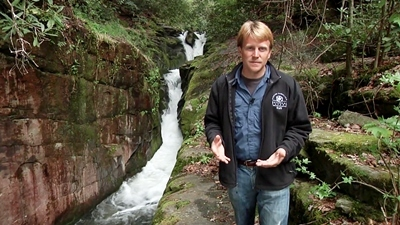1st Sunday of the Month Expeditions with naturalist Patrick McMillan. 10am - 2pm \$75 per person.

# Jocassee Lake Tours

## First Sunday Expeditions with Patrick McMillan

Something completely new and different from Jocassee Lake Tours. Join Emmy Award winning television host and naturalist Patrick McMillan for an unforgettable adventure on Lake Jocassee on the first Sunday of each month. Towering majestic waterfalls, birdlife, incredible scenery and of course the abundance of wildflowers are woven together by the experience and artful storytelling of Dr. McMillan.

## Sunset and Moon Rising Lake Tours

Have you ever sat in the middle of 9,000 acres of sparkling diamonds? That's how it feels to be on Lake Jocassee on a warm September evening as the setting sun kisses the mountaintops. Lake Jocassee Tours offers opportunities to witness the incredible beauty of a late day on the lake.

 table div table+table+table+table+table+table+table+table+table+table+table+table+table+table+table+table+table+table+table+table+table+table+table+table+table+table div table{width:100%;padding:0}table div table+table+table+table+table+table+table+table+table+table+table+table+table+table+table+table+table+table+table+table+table+table+table+table+table+table div table img{width:96.23%;padding:0;float:none}table div table+table+table+table+table+table+table+table+table+table+table+table+table+table+table+table+table+table+table+table+table+table+table+table+table+table div table td{width:100%;padding:0 1.88% 18px}/* styles */## MUSIC & THEATERThursday night street parties in downtown Seneca on Ram Cat Alley
 table.module-28{width:91.13%;padding:0}table div table+table+table+table+table+table+table+table+table+table+table+table+table+table+table+table+table+table+table+table+table+table+table+table+table+table+table+table+table div table{width:91.13%;float:none;margin-left:auto;margin-right:auto;padding:0}table div table+table+table+table+table+table+table+table+table+table+table+table+table+table+table+table+table+table+table+table+table+table+table+table+table+table+table+table+table div table a{border:0 none;text-decoration:none}table div table+table+table+table+table+table+table+table+table+table+table+table+table+table+table+table+table+table+table+table+table+table+table+table+table+table+table+table+table div table img{width:100%!important;border:0 none;text-decoration:none}table div table+table+table+table+table+table+table+table+table+table+table+table+table+table+table+table+table+table+table+table+table+table+table+table+table+table+table+table+table div table td{width:100%;padding:0}/* styles */

# Walhalla Civic AuditoriumANDY OFFUTT IRWIN (Story Teller/Comedian) September 15thRICK WADE'S TRIPLE TRIBUTE (to Elvis, Conway & Kenny) Friday, September 22nd
 table div table+table+table+table+table+table+table+table+table+table+table+table+table+table+table+table+table+table+table+table+table+table+table+table+table+table+table+table+table+table+table+table div table{width:100%;padding:0}table div table+table+table+table+table+table+table+table+table+table+table+table+table+table+table+table+table+table+table+table+table+table+table+table+table+table+table+table+table+table+table+table div table img{width:96.23%;padding:0;float:none}table div table+table+table+table+table+table+table+table+table+table+table+table+table+table+table+table+table+table+table+table+table+table+table+table+table+table+table+table+table+table+table+table div table td{width:100%;padding:0 1.88% 18px}/* styles */## FARMERS MARKETSHwy. 123 across from Blue Ridge Electric. SATURDAYS 8:30am-12:30pm.At Norton Thompson Park Downtown Seneca. TUES. THURS. SAT. 7am - noon.Main St. next to Arby's. MON. 7-10am & THURS. 4-7pm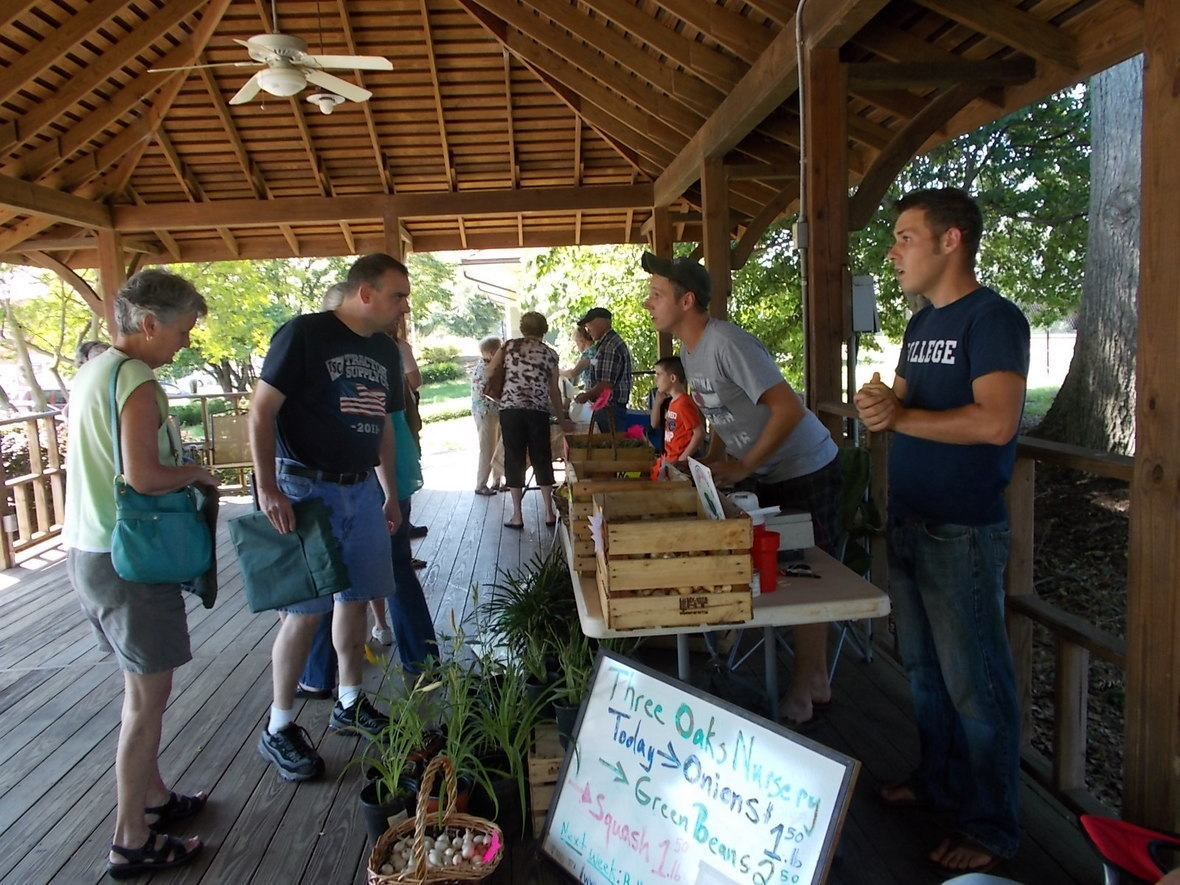Westminster Depot Gazebo TUESDAYS 4-7pm
 table div table+table+table+table+table+table+table+table+table+table+table+table+table+table+table+table+table+table+table+table+table+table+table+table+table+table+table+table+table+table+table+table+table+table+table div table{width:100%;padding:0}table div table+table+table+table+table+table+table+table+table+table+table+table+table+table+table+table+table+table+table+table+table+table+table+table+table+table+table+table+table+table+table+table+table+table+table div table img{width:96.23%;padding:0;float:none}table div table+table+table+table+table+table+table+table+table+table+table+table+table+table+table+table+table+table+table+table+table+table+table+table+table+table+table+table+table+table+table+table+table+table+table div table td{width:100%;padding:0 1.88% 18px}/* styles */Looking for a quick reference for things to do in Oconee County? SC Mountain Lakes website is your source for: Lodging, Dining, History, Happenings and more!